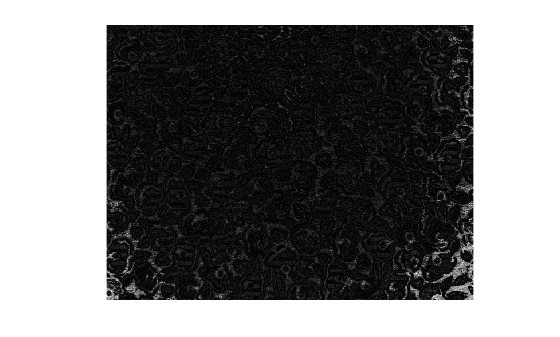# imcolordiff

Color difference based on CIE94 or CIE2000 standard

## Syntax

``dE = imcolordiff(I1,I2)``
``dE = imcolordiff(I1,I2,Name,Value)``

## Description

example

````dE = imcolordiff(I1,I2)` calculates the color difference between two RGB images or color maps using the CIE94 standard.```

example

````dE = imcolordiff(I1,I2,Name,Value)` specifies additional aspects of the computation, such as the input color space and the CIE standard, using one or more name-value pair arguments.```

## Examples

collapse all

Read a color image into the workspace.

```I1 = imread('peppers.png'); imshow(I1)```Alter the local color contrast in the image.

```I2 = localcontrast(I1); imshow(I2)```Calculate the color difference of the images using the default color standard, CIE94.

`dE = imcolordiff(I1,I2);`

Display the color difference as an image. Scale the display range to use the full range of pixel values in `dE`.

`imshow(dE,[])`Read and display an image of tissue stained with hemotoxylin and eosin (H&E).

```he = imread('hestain.png'); imshow(he)```Convert the image to the L*a*b* color space.

`lab = rgb2lab(he);`

Make a copy of the image, then increase the signal of the a* channel. Red tones in the image become more saturated while the image overall brightness and the blue tones are unchanged.

```lab2 = lab; scaleFactor = 1.1; lab2(:,:,2) = scaleFactor*lab(:,:,2);```

Calculate the color difference of the original and enhanced image in the L*a*b* color space.

`dE = imcolordiff(lab,lab2,'isInputLab',true);`

Display the color difference as an image. Scale the display range to match the range of pixel values in `dE`. Bright regions indicate the greatest color difference and correspond with the pink regions of tissue.

`imshow(dE,[])`Specify two RGB color values.

```pureRed = uint8([255,0,0]); darkRed = uint8([255,10,50]);```

Calculate the color difference of the colors using the CIEDE2000 standard.

`dE = imcolordiff(pureRed,darkRed,"Standard","CIEDE2000")`
```dE = single 7.4449 ```

Read and display an RGB image of fabric.

```fabric = imread('fabric.png'); imshow(fabric)```Simulate a second image of fabric by altering the local color contrast in the image.

```fabric2 = localcontrast(fabric); imshow(fabric2)```Calculate the color difference of the two images using the CIEDE2000 standard. Specify a luminance coefficient and K1 and K2 weighting factors appropriate for textiles.

```dE = imcolordiff(fabric,fabric2,'Standard','CIEDE2000', ... 'kL',2,'K1',0.048,'K2',0.014);```

Display the color difference. Scale the display range to the full range of pixel values in `dE`.

`imshow(dE,[])`## Input Arguments

collapse all

First set of color data, specified as an m-by-n-by-3 numeric array representing an image or a c-by-3 numeric matrix representing a set of c colors. `I1` and `I2` must be the same size with values in the same color space.

By default, the `imcolordiff` function interprets the color data as RGB color values. To calculate the color difference in the L*a*b* color space, specify the '`isInputLab`' argument as `true`. L*a*b* color values can be of data type `single` or `double` only.

Data Types: `single` | `double` | `uint8` | `uint16`

Second set of color data, specified as an m-by-n-by-3 numeric array representing an image or a c-by-3 numeric matrix representing a set of c colors. `I1` and `I2` must be the same size with values in the same color space.

By default, `imcolordiff` interprets the color data as RGB color values. To calculate the difference of colors in the L*a*b* color space, specify the '`isInputLab`' argument as `true`. L*a*b* color values can be of data type `single` or `double` only.

Data Types: `single` | `double` | `uint8` | `uint16`

### Name-Value Arguments

Specify optional pairs of arguments as `Name1=Value1,...,NameN=ValueN`, where `Name` is the argument name and `Value` is the corresponding value. Name-value arguments must appear after other arguments, but the order of the pairs does not matter.

Before R2021a, use commas to separate each name and value, and enclose `Name` in quotes.

Example: `'Standard',"CIEDE2000"` calculates the color difference between two RGB images using the CIEDE2000 standard.

CIE standard used to compute the color difference value, specified as the comma-separated pair consisting of `'Standard'` and one of these values:

ValueDescription
`"CIE94"`The CIE94 standard. This standard improves the perceptual non-uniformities of the CIE76 standard implemented in the `deltaE` function.
`"CIEDE2000"`The CIEDE2000 standard. This standard further improves the perceptual uniformity through five additional corrections: a hue rotation term, compensation for neutral colors, and compensation for lightness, chroma, and hue.

Data Types: `char` | `string`

Color values are in the L*a*b* color space, specified as the comma-separated pair consisting of `'isInputLab'` and a numeric or logical `0` (`false`) or `1` (`true`).

Luminance coefficient, specified as the comma-separated pair consisting of `'kL'` and a numeric scalar. The luminance coefficient is typically `1` for applications in graphic arts and `2` for applications in textiles.

K1 weighting factor, specified as the comma-separated pair consisting of `'K1'` and a numeric scalar. The K1 weighting factor is typically `0.045` for applications in graphic arts and `0.048` for applications in textiles.

K2 weighting factor, specified as the comma-separated pair consisting of `'K2'` and a numeric scalar. The K2 weighting factor is typically `0.015` for applications in graphic arts and `0.014` for applications in textiles.

## Output Arguments

collapse all

Color difference (delta E), returned as one of the following.

• An m-by-n matrix when the input color data `I1` and `I2` represent images

• A c-element column vector when `I1` and `I2` represent a set of c colors

If `I1` or `I2` is of data type `double`, then `dE` is of data type `double`. Otherwise, `dE` is of data type `single`.

Data Types: `single` | `double`

## Tips

• To calculate color differences following the CIE76 standard, use the `deltaE` function. This function is faster than the `imcolordiff` function, but less precise.

 Sharma, Gaurav, Wencheng Wu, and Edul N. Dalal, "The CIEDE2000 Color-Difference Formula: Implementation Notes, Supplementary Test Data, and Mathematical Observations". Color Research and Application 30, no. 1 (February 2005): 21–30. https://doi.org/10.1002/col.20070.